Subsections

# Debye Model of Crystals

## Idea

Werepresented each atom as a harmonic oscillator. It did not work.

Let us represent our system as a set of 3N oscillators, but choose them differently, including collective motion.

## One-Dimensional Crystal

We have N particles with masses m on springs k:Equation of motion: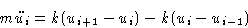(3)
or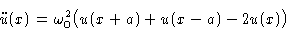(4)
Periodic boundary conditions: u1=uN+1. Sound velocity: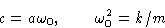(5)

## Modes

All ui are entangled! To disentangle them--use a trick: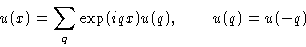(6)
What can we say about q? We want u(Na)=u(0), or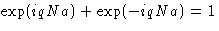for all q. This means that,or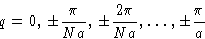Physics: we represented deviation as sum of waves: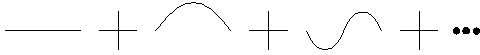These waves are called modes

## Dispersion Equation. Meaning of Phonons

Substitute (6) into (4):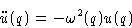with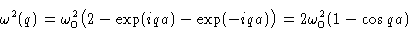For small q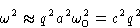Solution: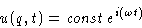In real space we obtain sum of terms proportional to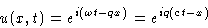This describes sound waves propagating with velocity c (that is why we claimed that c is sound velocity)

Equation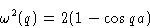(7)
is called dispersion equation. Each q corresponds to one normal mode or one kind of phonons--in other words, to a sound wave.

Why Debye model is better than Einstein model: since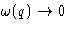at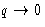, some phonons are excited even at low temperatures!

## Heat Capacity for 1D Crystal

We obtained N independent oscillators (modes!) with frequenciesEach gives a contribution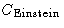to the heat capacity. Total contribution: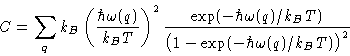If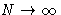--integration instead of summation. We have N values for q in the interval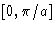--so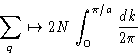## 3D Crystals

What's new for 3D?

1.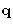is now vector--instead of dq we must integrate over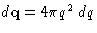, q2=qx2+qy2+qz2.
2.
There are three waves for every: two transversal and one longitudinal

Instead of integrating by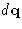we integrate by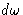. Number of modes: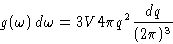Limits: lower limit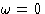, upper limit should be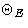, but we used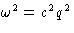instead of exact equation (7), so we correct this by taking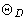instead: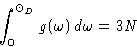Result (for simple lattice!):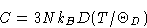with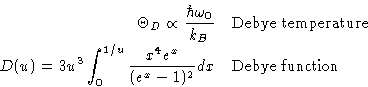(8)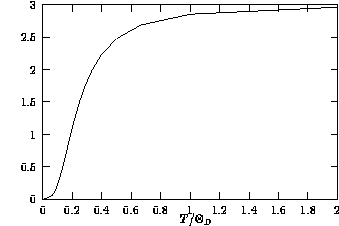High temperatures:
for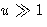upper limit in the integral in (8) is small (1/u),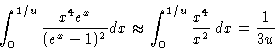and C=3NkB
Low temperatures:
for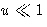integral is constant, and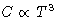. This is an example of a scaling, made by a classic of physics as early as 1912!Next: Quiz Up: Solid State Previous: Einstein Model of Crystals

© 1997 Boris Veytsman and Michael Kotelyanskii
Wed Oct 1 00:45:35 EDT 1997